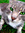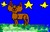# ♥♥♥Cinderpelt Lovers♥♥♥((Organization in Progress)) discussion

10 views
Group Monthly Goals > March2010's Group Member Goal

message 1: by SpottiPup407, Loves Graystripe (new)

This month, our goal is to finally get forty members by the end of March!And I hope you guys break it!Advertise, invite, and tell your friends to invite their friends!:PBut I hope you all break it!

Thanks!

Dapplekit

message 4: by Syeira-la, "They say there's no such place... as Paradise..." (new)

Hiya! Welcome to the group.

message 5: by Sephiroth, I BEG TO DREAM AND DIFFER FROM THE HOLLOW LIES...ON HOLIDAY!! (new)

Lol, hiya Grayfur. You can jump into the RP anytime, or you can ask Dapplekit if you can create discussion topics about Warriors. ^^

message 6: by SpottiPup407, Loves Graystripe (new)

Hey, Grayfur!Welcome yo Cinderpelt Lovers!And thanks for joining!:)☺☺☺☺

message 7: by (new)

message 8: by SpottiPup407, Loves Graystripe (new)

Awesome!!!!!!!!!!!!!Thanks to you all who invited!!!!!!!☺☺☺☺☺☺☺☺☺☺☺

message 10: by Syeira-la, "They say there's no such place... as Paradise..." (new)

Woooooah Madhins--300!? Mine can only be people I rp with almost every day, haha. So I only have 20 or so.

message 11: by (last edited Mar 17, 2010 08:09PM) (new)Lol, I've made a lot of friends through my good-readen time... Most of 'em don't get on much anymore but the friendship was good while it lasted. XD

message 12: by Sephiroth, I BEG TO DREAM AND DIFFER FROM THE HOLLOW LIES...ON HOLIDAY!! (new)

wow, i only have 96 friends, and i thought that was alot...*gives 'Most Freidns on Goodreads' prize to Madhins*

message 13: by SpottiPup407, Loves Graystripe (new)

Did you invite all them, Madhins?

message 14: by Sephiroth, I BEG TO DREAM AND DIFFER FROM THE HOLLOW LIES...ON HOLIDAY!! (new)

hiya, dapplekit!

message 15: by SpottiPup407, Loves Graystripe (new)

message 16: by Sephiroth, I BEG TO DREAM AND DIFFER FROM THE HOLLOW LIES...ON HOLIDAY!! (new)

message 17: by SpottiPup407, Loves Graystripe (new)

:DI can't believe the group has forty-one members!I should've made it smaller earlier!:D

message 18: by Sephiroth, I BEG TO DREAM AND DIFFER FROM THE HOLLOW LIES...ON HOLIDAY!! (new)

lol, yeah. now it is less confusing!

message 19: by SpottiPup407, Loves Graystripe (new)

:D Can you invite every one of your friends to join????

message 20: by Sephiroth, I BEG TO DREAM AND DIFFER FROM THE HOLLOW LIES...ON HOLIDAY!! (new)

message 21: by SpottiPup407, Loves Graystripe (new)

Yay, thanks!!!

message 22: by Sephiroth, I BEG TO DREAM AND DIFFER FROM THE HOLLOW LIES...ON HOLIDAY!! (new)

np, i g2g

message 23: by SpottiPup407, Loves Graystripe (new)

Aww....Bye!

message 24: by (new)

message 25: by SpottiPup407, Loves Graystripe (new)

Yay1!!:):):):):):):):):):):):):):):):):):):):):):):):):):):):):):):):):):):):):):):):):):):):):):):):):):):):):):):):):):):):):):):):):):):):):):):):):):):):):):):):):):):):):):):):):):):):):):):):):):):):):):):):):):):):):):):):):):):):):):):):):):):):):):):):):):):):):):):):):):):):):):):):):):):):):):):):):):):):):):):):):):):):):):):):):):):):):):):):):):):):):):):):):):):):):):):):):):):):):):):):):):):):):):):):):):):):):):):):):):):):):):):):):):):):):):):):):):):):):):):):):):):):):):):):):):):):):):):):):):):):):):):):):):):):):):):):):):):):):):):):):):):):):):):):):):):):):):):):):):):):):):):):):):):):):):):):):):):):):):):):):):):):):):):):):):):):):):):):):):):):):):):):):):):):):):):):):):):):):):):):):):):):):):):):):):):):):):):):):):):):):):):):):):):):):):):):):):):):):):):):):):):):):):):):):):):):):):):):):):):):):):):):):):):):):):):):):):):):):):):):):):):):):):):):):):):):):):):):):):):):):):):):):):):):):):):):):):):):):):):):):):):):):):):):):):):):):):):):):):):):):):):):):):):):):):):):):):):):):):):):):):):):):):):):):):):):):):):):):):):):):):):):):):):):):):):):):):):):):):):):):):):):):):):):):):):):):):):):):):):):):):):):):):):):):):):):):):):):):):):):):):):):):):):):):):):):):):):):):):):):):):):):):):):):):):):):):):):):):):):):):):):):):):):):):):):):):):):):):):):):):):):):):):):):):):):):):):):):):):):):):):):):):):):):):):):):):):):):):):):):):):):):):):):):):):):):):):):):):):):):):):):):):):):):):):):):):):):):):):):):):):):):):):):):):):):):):):):):):):):):):):):):):):):):):):):):):):):):):):):):):):):):):):):):):):):):):):):):):):):):):):):):):):):):):):):):):):):):):):):):):):):):):):):):):):):):):):):):):):):):):):):):):):):):):):):):):):):):):):):):):):):):):):):):):):):):):):):):):):):):):):):):):):):):):):):):):):):):):):):):):):):):):):):):):):):):):):):):):):):):):):):):):):):):):):):):):):):):):):):):):):):):):):):):):):):):):):):):):):):):):):):):):):):):):):):):):):):):):):):):):):):):):):):):):):):):):):):):):):):):):):):):):):):):):):):):):):):):):):):):):):):):):):):):):):):):):):):):):):):):):):):):):):):):):):):):):):):):):):):):):):):):):):):):):):):):):):):):):):):):):):):):):):):):):):):):):):):):):):):):):):):):):):):):):):):):):):):):):):):):):):):):):):):):):):):):):):):):):):):):):):):):):):):):):):):):):):):):):):):):):):):):):):):):):):):):):):):):):):):):):):):):):):):):):):):):):):):):):):):):):):):):):):):):):):):):):):):):):):):):):):):):):):):):):):):):):):):):):):):):):):):):):):):):):):):):):):):):):):):):):):):):):):):):):):):):):):):):):):):):):):):):):):):):):):):):):):):):):):):):):):):):):):):):):):):):):):):):):):):):):):):):):):):):):):):):):):):):):):):):):):):):):):):):):):):):):):):):):):):):):):):):):):):):):):):):):):):):):):):):):):):):):):):):):):):):):):):):):):):):):):):):):):):):):):):):):):):):):):):):):):):):):):):):):):):):):):):):):):):):):):):):):):):):):):):):):):):):):):):):):):):):):):):):):):):):):):):):):):):):):):):):):):):):):):):):):):):):):):):):):):):):):):):):):):):):):):):):):):):):):):):):):):):):):):):):):):):):):):):):):):):):):):):):):):):):):):):):):):):):):):):):):):):):):):):):):):):):):):):):):):):):):):):):):):):):):):):):):):):):):):):):):):):):):):):):):):):):):):):):):):):):):):):):):):):):):):):):):):):):):):):):):):):):):):):):):):):):):):):):):):):):):):):):):):):):):):):):):):):):) :):):):):):):):):):):):):):):):):):):):):):):):):):):):):):):):):):):):):):):):):):):):):):):):):):):):):):):):):):):):):):):):):):):):):):):):):):):):):):):):):):):):):):):):):):):):):):):):):):):):):):):):):):):):):):):):):):):):):):):):):):):):):):):):):):):):):):):):):):):):):):):):):):):):):):):):):):):):):):):):):):):):):):):):):):):):):):):):):):):):):):):):):):):):):):):):):):):):):):):):):):):):):):):):):):):):):):):):):):):):):):):):):):):):):):):):):):):):):):):):):):):):):):):):):):):):):):):):):):):):):):):):):):):):):):):):):):):):):):):):):):):):):):):):):):):):):):):):):):):):):):):):):):):):):):):):):):):):):):):):):):):):):):):):):):):):):):):):):):):):):):):):):):):):):):):):):):):):):):):):):):):):):):):):):):):):):):):):):):):):):):):):):):):):):):):):):):):):):):):):):):):):):):):):):):):):):):):):):):):):):):):):):):):):):):):):):):):):):):):):):):):):):):):):):):):):):):):):):):):):):):):):):):):):):):):):):):):):):):):):):):):):):):):):):):):):):):):):):):):):):):):):):):):):):):):):):):):):):):):):):):):):):):):):):):):):):):):):):):):):):):):):):):):):):):):):):):):):):):):):):):):):):):):):):):):):):):):):):):):):):):):):):):):):):):):):):):):):):):):):):):):):):):):):):):):):):):):):):):):):):):):):):):):):):):):):):):):):):):):):):):):):):):):):):):):):):):):):):):):):):):):):):):):):):):):):)

message 26: by [deleted user] (new)

cool